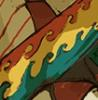# "position by uv value" from hou.surface and hou.face modules.

## Recommended Posts

Hi, I am trying to make a point deformer and I am stucked in a step.

Here is my plans

1. copy all the points that I want to deform to the polygon object so they are 1 object using hou.geo.createPoint in python.
2. get uv attributes from the object
3. copy the uv attributes of first frame so it will contain the static uv value while the object is deforming.
4. get position value from the uv and fetch the output position value to the points that I want to deform but it seems like from the uv, it's not getting the right position.

Can anybody take a look inside of this file and what step I am missing?

point copy to object python script

`node = hou.pwd()geo = node.geometry()geo2 = node.inputs().geometry()ptGrp = geo.createPointGroup("ptGrp")for point in geo2.points():pos = point.attribValue("P")pt = geo.createPoint()pt.setAttribValue("P" , pos)ptGrp.add(pt)[/CODE]`

[b]get position by uv and fetch to the original points script[/b]

[CODE]
node = hou.pwd()
geoPdef = hou.pwd().geometry()
geoAnim = node.inputs().geometry()

fPtGrp = geoAnim.findPointGroup("ptGrp")
ptsGrp = fPtGrp.points()
i = 0
for point in geoPdef.points():
pt = ptsGrp[i]
ppos = pt.attribValue("P")
nprim = geoAnim.nearestPrim(ppos)
u = pt.attribValue("uv")
v = pt.attribValue("uv")
pos = nprim.positionAt(u)
point.setAttribValue("P" , pos)
i += 1 [/CODE]

test.hip

Edited by cloudfx

## Create an account

Register a new account